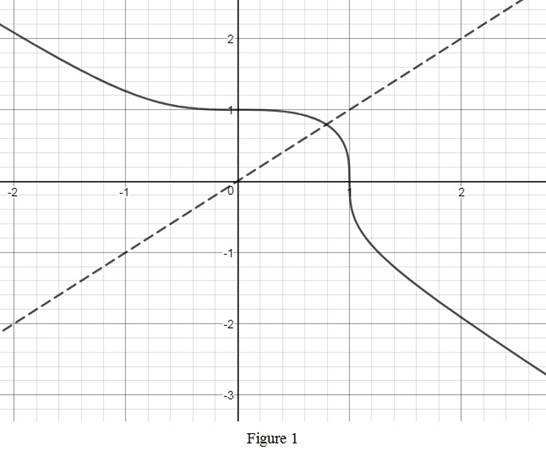# The inverse of the function g ( x ) = 1 − x 3 3 ; how g − 1 is related to g .### Single Variable Calculus: Concepts...

4th Edition
James Stewart
Publisher: Cengage Learning
ISBN: 9781337687805### Single Variable Calculus: Concepts...

4th Edition
James Stewart
Publisher: Cengage Learning
ISBN: 9781337687805

#### Solutions

Chapter 1.6, Problem 32E

(a)

To determine

## To find: The inverse of the function g(x)=1−x33 ; how g−1 is related to g.

Expert Solution

The inverse of the function g(x)=1x33 is g1(x)=1x33 and g1=g .

### Explanation of Solution

Let the given function as y=(1x3)13 .

Solve this equation for x as follows.

y3=1x31y3=x3x=1y33

Interchange x and y and obtain the inverse function, y=1x33 .

Thus, the required inverse function is g1(x)=1x33 .

Observe that the given function and its inverse are the same. That is, g1=g .

(b)

To determine

Expert Solution

### Explanation of Solution

Use online graphing calculator and draw the graph of g(x)=1x33 as shown below in Figure 1.From Figure 1, it is observed that the graph of g(x)=1x33 is a reflection of the graph g1(x)=1x33 about the line y=x . Notice that the graphs of g1(x) and g(x) are same.

### Have a homework question?

Subscribe to bartleby learn! Ask subject matter experts 30 homework questions each month. Plus, you’ll have access to millions of step-by-step textbook answers!